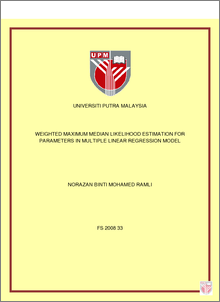# Weighted Maximum Median Likelihood Estimation For Parameters In Multiple Linear Regression Model

## Citation

Mohamed Ramli, Norazan (2008) Weighted Maximum Median Likelihood Estimation For Parameters In Multiple Linear Regression Model. PhD thesis, Universiti Putra Malaysia.

## Abstract

The performance of the Maximum Median Likelihood Estimator (MML) proposed by Hao (1992) is very inconsistent and sensitive to outliers, which results in biased parameter estimates. We propose Weighted Maximum Median Likelihood (WMML) estimators as alternatives. The basic idea in the WMML estimation is to isolate outliers from the majority of the observations and use only a certain number of fittest observations to estimate parameters. We study in details the performance of the WMML estimators in real and simulated data sets. The WMML estimates are consistent and can be as good as the MLE estimates in outlier free data sets and more efficient for data sets with multiple outliers than the MLE and MML estimates. The research also develops a diagnostic method for the identification of high-leverage points. We realize that often their presence in data set gives adverse effect on the inference. We propose Diagnostic-Robust Generalized Potentials (DRGP) technique as an alternative approach that performs well relative to current techniques. The WMML estimators also function as indirect methods for identifying multiple outliers in data sets. Visual analysis of the WMML estimates shows that the estimators can be a reliable method to identify multiple outliers in linear regression. We also propose Transformed Both Sides (TBS) Robust Based estimators, namely the TBS-WMML1 Based estimator, the TBS-WMML2 Based estimator and the TBS-WMML3 Based estimator for data sets with problems of outliers and non-constant variance error terms. The problem of non-constant variance error terms is also known as heteroscedastic problem. To induce homoscedasticity for data sets with outliers, TBS-Robust Based estimators are used. The resulting estimates are expected to have constant variance and the resulting model is called the TBS model with constant variance error terms. Our analysis shows that the TBS-Robust Based estimators provide estimates with lower variance than the MLE and the MML methods when both problems of outliers and non-constant variance errors exist. The thesis also checks the variability of the WMML estimators using bootstrap procedures. Current bootstrap procedures such as Fixed-x Resampling, Random-x Resampling and Diagnostic Before Bootstrap are not robust to outliers. To accommodate this problem we propose a new bootstrap procedure, which we call as Weighted Bootstrap with Probability (WBP). In the WBP procedure, outlying observations are attributed with low probabilities and consequently with low chances of being selected in the re-sampling process. Simulation results show that in most instances the WBP is more robust against a given number of arbitrary outliers than the current bootstrap procedures.Preview
PDF
FS_2008_33.pdfView Item